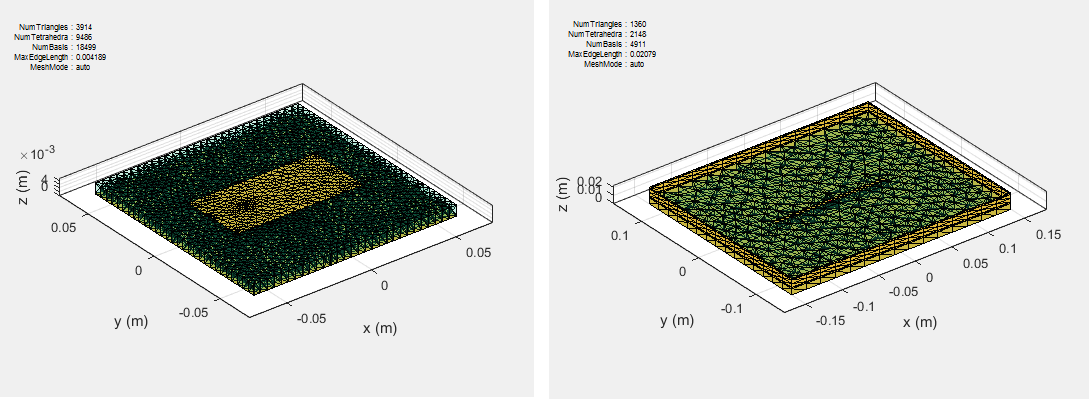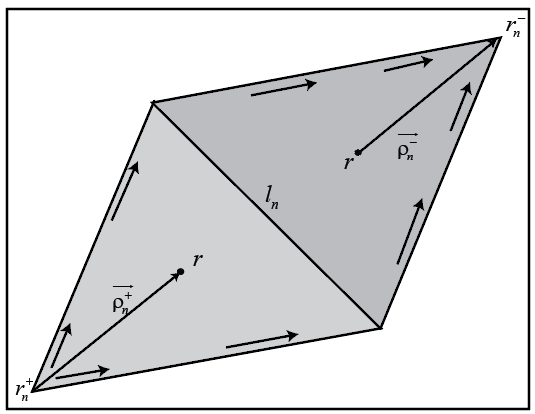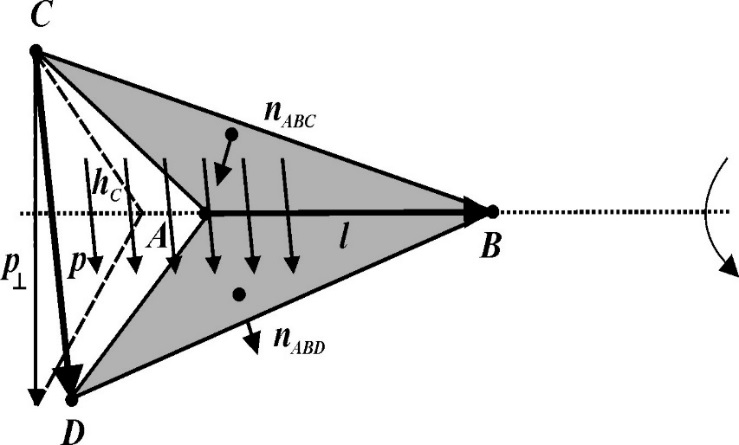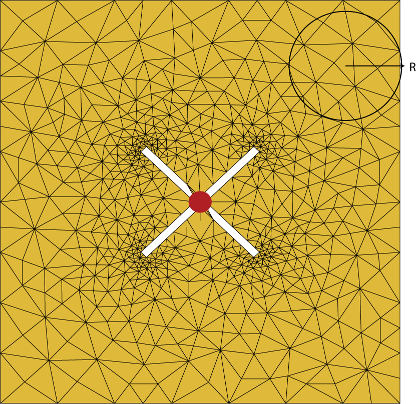## Method of Moments Solver for Metal and Dielectric Structures

Method of Moments computation technique for metal and dielectrics in a PCB.

A PCB consists of a metal part and a dielectric part. The first step in the computational solution of electromagnetic problems is to discretize Maxwell's equations. The process results in this matrix-vector system:

`$V=ZI$`
• V — Applied voltage vector. This signal can be voltage or power applied to the antenna or an incident signal falling at the input port of the PCB component.

• I — Current vector that represents current on the PCB component surface.

• Z — Interaction matrix or impedance matrix that relates V to I. For calculating the interaction matrix, the effect of metal and dielectric parts iof the PCB antenna are taken separately.

RF PCB Toolbox™ uses method of moments (MoM) to calculate the interaction matrix and solve system equations.

### MoM Formulation

The MoM formulation is split into three parts.

#### Discretization of Metals and Dielectrics

Discretization enables the formulation from the continuous domain to the discrete domain. This step is called meshing. In the MoM formulation, the metal surface of the PCB is meshed into triangles and the dielectric volume is meshed into tetrahedrons.#### Basis Functions

Metals.   To calculate the surface currents on the PCB, first define basis functions. RF PCB Toolbox uses Rao-Wilton-Glisson (RWG)  basis functions. The arrows show the direction of current flow.The basis function includes a pair of adjacent (not necessarily coplanar) triangles and resembles a small spatial dipole with linear current distribution. Each triangle is associated with a positive or negative charge.

For any two triangle patches, ${t}_{n}^{+}$ and ${t}_{n}^{-}$, having areas ${A}_{n}^{+}$ and ${A}_{n}^{-}$, and sharing common edge ${l}_{n}$, the basis function is

`$\begin{array}{l}{\stackrel{\to }{f}}_{n}\left(\stackrel{\to }{r}\right)=\left\{\begin{array}{cc}\frac{{l}_{n}}{2{A}_{n}^{+}}{\stackrel{\to }{\rho }}_{n}^{+S},& \stackrel{\to }{r}\text{\hspace{0.17em}}\in \text{\hspace{0.17em}}{t}_{n}^{+}\\ \frac{{l}_{n}}{2{A}_{n}^{-}}{\stackrel{\to }{\rho }}_{n}^{-S},& \stackrel{\to }{r}\text{\hspace{0.17em}}\in \text{\hspace{0.17em}}{t}_{n}^{-}\end{array}\text{ }\\ \end{array}$`
• ${\stackrel{\to }{\rho }}_{n}^{+}=\stackrel{\to }{r}-{\stackrel{\to }{r}}_{n}^{+}$ — Vector drawn from the free vertex of triangle ${t}_{n}^{+}$ to observation point $\stackrel{\to }{r}$

• ${\stackrel{\to }{\rho }}_{n}^{-}={\stackrel{\to }{r}}_{n}^{+}-\stackrel{\to }{r}$ — Vector drawn from the observation point to the free vertex of the triangle ${t}_{n}^{-}$

and

`$\nabla \cdot {\stackrel{\to }{f}}_{n}\left(\stackrel{\to }{r}\right)=\left\{\begin{array}{cc}\frac{{l}_{n}}{{A}_{n}^{+}},& \stackrel{\to }{r}\text{\hspace{0.17em}}\in \text{\hspace{0.17em}}{t}_{n}^{+}\\ -\frac{{l}_{n}}{{A}_{n}^{-}},& \stackrel{\to }{r}\text{\hspace{0.17em}}\in \text{\hspace{0.17em}}{t}_{n}^{-}\end{array}$`

The basis function is zero outside the two adjacent triangles ${t}_{n}^{+}$ and ${t}_{n}^{-}$. The RWG vector basis function is linear and has no flux (no normal component) through its boundary.

Dielectrics.  Basis functions are used to represent unknown quantities. In the case of a PCB component, the unknown quantities are the surface current on the metal structure and flux density due to dielectric volume. RF PCB Toolbox uses Rao-Wilton-Glisson (RWG)  basis functions.

For the dielectric volume, RF PCB Toolbox uses a zeroth order edge basis function to model the flux density.The figure shows an edge-based basis function. The vector variation is perpendicular to the base edge AB (or $\overline{l}$). The vector of the edge CD (or $\overline{p}$) defines the basis function. Within a tetrahedron, the basis function is a constant field given by

`$\stackrel{\to }{f}=c\stackrel{\to }{p}$`

• c – normalization coefficient.

• p – vector of the edge defining the basis function.

#### Interaction Matrix

Metals.  The interaction matrix is a complex dense symmetric matrix. It is a square N-by-N matrix, where N is the number of basis functions, that is, the number of interior edges in the structure. A typical interaction matrix for a structure with 256 basis functions is shown:To fill out the interaction matrix, calculate the free-space Green's function between all basis functions on the PCB surface. The final interaction matrix equations are:

`${Z}_{mn}=\left(\frac{j\omega \mu }{4\pi }\right)\underset{S}{\int }\underset{S}{\int }{\stackrel{\to }{f}}_{m}\left(\stackrel{\to }{r}\right).{\stackrel{\to }{f}}_{m}\left(\stackrel{\to }{{r}^{\prime }}\right)gd\stackrel{\to }{{r}^{\prime }}d\stackrel{\to }{r}-\left(\frac{j}{4\pi \omega \epsilon }\right)\underset{S}{\int }\underset{S}{\int }\left(\nabla .{\stackrel{\to }{f}}_{m}\right)\left(\nabla .{\stackrel{\to }{f}}_{m}\right)gd\stackrel{\to }{{r}^{\prime }}d\stackrel{\to }{r}$`

where

• $g\left(\stackrel{\to }{r},\stackrel{\to }{{r}^{\prime }}\right)=\frac{\mathrm{exp}\left(-jk|\stackrel{\to }{r}-\stackrel{\to }{{r}^{\prime }}|\right)}{|\stackrel{\to }{r}-\stackrel{\to }{{r}^{\prime }}|}$ — Free-space Green's function

To calculate the interaction matrix, excite the PCB by a voltage of 1 V at the input port. So the voltage vector has zero values everywhere except at the feeding edge. Solve the system of equations to calculate the unknown currents. Once you determine the unknown currents, you can calculate the field and surface properties of the PCB.

Dielectrics.  The interaction matrix is a complex dense symmetric matrix. To fill out the interaction matrix, calculate the free-space Green's function between all the basis functions on the PCB surface. The final interaction matrix equations are:

• `ZMM` – metal to metal interaction. For a pure metal structure, you only calculate this symmetric square matrix.

`${Z}_{mn}^{MM}=\left(\frac{j\omega \mu }{4\pi }\right)\underset{S}{\int }\underset{S}{\int }{\stackrel{\to }{f}}^{M}{}_{m}\left(\stackrel{\to }{r}\right).{\stackrel{\to }{f}}^{M}{}_{n}\left(\stackrel{\to }{{r}^{\prime }}\right)gd\stackrel{\to }{{r}^{\prime }}d\stackrel{\to }{r}-\left(\frac{j}{4\pi \omega \epsilon }\right)\underset{S}{\int }\underset{S}{\int }\left(\nabla .{\stackrel{\to }{f}}^{M}{}_{m}\right)\left(\nabla .{\stackrel{\to }{f}}^{M}{}_{n}\right)gd\stackrel{\to }{{r}^{\prime }}d\stackrel{\to }{r}$`
• `ZDD` – dielectric to dielectric interaction. For pure dielectric structures, you only calculate this symmetric square matrix.

`$\begin{array}{l}{\stackrel{^}{Z}}_{mn}^{DD}=\sum _{p-1}^{P}\sum _{{p}^{\prime }-1}^{{P}^{\prime }}\frac{{K}_{p}}{{\stackrel{^}{\epsilon }}_{p}}\underset{{V}_{D}}{\int }{\stackrel{\to }{f}}_{mp}\left(\stackrel{\to }{r}\right)\cdot \text{ }\text{ }{\stackrel{\to }{f}}_{n{p}^{\prime }}\left(\stackrel{\to }{r}\right)d\stackrel{\to }{r}\\ \text{\hspace{0.17em}}\text{ }\text{\hspace{0.17em}}\text{ }-\frac{{\omega }^{2}{\mu }_{0}}{4\pi }\sum _{p-1}^{P}\sum _{{p}^{\prime }-1}^{{P}^{\prime }}{K}_{p}{K}_{{p}^{\prime }}\underset{{V}_{D}}{\int }\underset{{V}_{{D}^{\prime }}}{\int }g\left(\stackrel{\to }{r},\stackrel{\to }{{r}^{\prime }}\right){\stackrel{\to }{f}}_{mp}\left(\stackrel{\to }{r}\right)\cdot {\stackrel{\to }{f}}_{n{p}^{\prime }}\left(\stackrel{\to }{{r}^{\prime }}\right)d\stackrel{\to }{r}d\stackrel{\to }{{r}^{\prime }}\\ \text{ }\text{ }\text{\hspace{0.17em}}\text{\hspace{0.17em}}-\frac{1}{4\pi {\epsilon }_{0}}\sum _{q-1}^{Q}\sum _{{q}^{\prime }-1}^{{Q}^{\prime }}{\stackrel{^}{K}}_{q}{\stackrel{^}{K}}_{{q}^{\prime }}\underset{{\Omega }_{q}}{\int }\underset{{\Omega }_{{q}^{\prime }}}{\int }g\left(\stackrel{\to }{r},\stackrel{\to }{{r}^{\prime }}\right){f}_{\perp mq}\left(\stackrel{\to }{r}\right){f}_{\perp n{q}^{\prime }}\left(\stackrel{\to }{{r}^{\prime }}\right){d}_{s}{d}_{{s}^{\prime }}\text{\hspace{0.17em}}\text{ }\text{ }\text{ }m,n=1,....,N\end{array}$`
• `ZMD` and `ZDM` – These matrices calculate the interaction between metal and dielectric. This matrix is not a symmetrical square matrix.

`$\begin{array}{l}{Z}_{mn}^{MD}=-\frac{{\omega }^{2}{\mu }_{0}}{4\pi }\sum _{p-1}^{2}\sum _{{p}^{\prime }-1}^{{P}^{\prime }}{K}_{p}\underset{t}{\int }\underset{{V}_{{D}^{\prime }}}{\int }{\stackrel{\to }{f}}_{n}^{M}\left(\stackrel{\to }{r}\right)\cdot \text{ }\text{ }{\stackrel{\to }{f}}_{m{p}^{\prime }}\left(\stackrel{\to }{{r}^{\prime }}\right)g\left(\stackrel{\to }{r},\stackrel{\to }{{r}^{\prime }}\right)d\stackrel{\to }{{r}^{\prime }}{d}_{s}\\ \text{\hspace{0.17em}}\text{ }\text{\hspace{0.17em}}\text{ }\text{\hspace{0.17em}}\text{\hspace{0.17em}}-\frac{1}{4\pi {\epsilon }_{0}}\sum _{p-1}^{2}\sum _{q-1}^{Q}{\stackrel{^}{K}}_{q}\underset{{t}_{D}}{\int }\underset{{\Omega }_{{q}^{\prime }}}{\int }\left({\nabla }_{s}\cdot {\stackrel{\to }{f}}_{n}^{M}\left(\stackrel{\to }{r}\right)\right){f}_{\perp mq}\left(\stackrel{\to }{{r}^{\prime }}\right)g\left(\stackrel{\to }{r},\stackrel{\to }{{r}^{\prime }}\right){d}_{{\Omega }^{\prime }}{d}_{s}\text{\hspace{0.17em}}\text{ }\text{ }\\ \text{ }\text{ }\text{\hspace{0.17em}}\text{ }m=1,....,{N}_{D};n=1,...,{N}_{M}\end{array}$`
`$\begin{array}{l}{Z}_{mn}^{DM}=-\frac{j\omega {\mu }_{0}}{4\pi }\sum _{p-1}^{2}\sum _{{p}^{\prime }-1}^{{P}^{\prime }}{K}_{{p}^{\prime }}\underset{{V}_{D}}{\int }\underset{{S}_{{D}^{\prime }}}{\int }{\stackrel{\to }{f}}_{np}\left(\stackrel{\to }{r}\right)\cdot {\stackrel{\to }{f}}_{m}^{M}\left(\stackrel{\to }{{r}^{\prime }}\right)g\left(\stackrel{\to }{r},\stackrel{\to }{{r}^{\prime }}\right){d}_{{s}^{\prime }}d\stackrel{\to }{r}\\ \text{\hspace{0.17em}}\text{ }\text{\hspace{0.17em}}\text{ }\text{\hspace{0.17em}}\text{\hspace{0.17em}}+\frac{1}{4\pi {\epsilon }_{0}\omega }\sum _{p-1}^{2}\sum _{q-1}^{Q}{\stackrel{^}{K}}_{q}\underset{{\Omega }_{q}}{\int }\underset{{S}_{{D}^{\prime }}}{\int }{f}_{\perp nq}\left(\stackrel{\to }{r}\right)\cdot \left({\nabla }_{s}\cdot {\stackrel{\to }{f}}_{m}^{M}\left(\stackrel{\to }{{r}^{\prime }}\right)\right)g\left(\stackrel{\to }{r},\stackrel{\to }{{r}^{\prime }}\right){d}_{{s}^{\prime }}{d}_{\Omega }\text{ }\text{ }\\ \text{ }\text{ }\text{\hspace{0.17em}}\text{ }m=1,....,{N}_{D};n=1,...,{N}_{M}\end{array}$`

where

• $g\left(\stackrel{\to }{r},\stackrel{\to }{{r}^{\prime }}\right)=\frac{\mathrm{exp}\left(-jkR\right)}{R},R=|\stackrel{\to }{r}-\stackrel{\to }{{r}^{\prime }}|$ is the free space Green's function.

• $K=\frac{{\stackrel{^}{\epsilon }}^{±}-{\epsilon }_{0}}{{\stackrel{^}{\epsilon }}^{±}}$ is the complex dielectric constant within every tetrahedron.

• ${\stackrel{^}{K}}_{q}={K}_{+}-{K}_{-}$ is the differential contrast on every face of the tetrahedron.

For a composite metal structure, you must calculate all four matrices.

### Neighbor Region

From the interaction matrix plot, you observe that the matrix is diagonally dominant. As you move further away from the diagonal, the magnitude of the terms decreases. This behavior is same as the Green's function behavior. The Green's function decreases as the distance between r and r' increases. Therefore, it is important to calculate the region on the diagonal and close to the diagonal accurately.This region on and around the diagonal is called neighbor region. The neighbor region is defined within a sphere of radius R, where R is in terms of triangle size. The size of a triangle is the maximum distance from the center of the triangle to any of its vertices. By default, R is twice the size of the triangle. For better accuracy, a higher-order integration scheme is used to calculate the integrals. The figure shows a typical interaction matrix for a metal structure `ZMM` with 256 basis functions.

The dielectric interaction matrix is also diagonally dominant. As you move further away from the diagonal, the magnitude of the terms decreases. This behavior is same as the Green's function behavior. The Green's function decreases as the distance between r and r' increases. Therefore, it is important to calculate the region on the diagonal and close to the diagonal accurately. For a dielectric the neighbor region is based on the average size of the tetrahedron.

### Singularity Extraction

Along the diagonal, r and r' are equal and defines Green's function becomes singular. To remove the singularity, extraction is performed on these terms.

`$\begin{array}{l}\underset{{t}_{p}}{\int }\underset{{t}_{q}}{\int }\left({\stackrel{\to }{\rho }}_{i}.{{\stackrel{\to }{\rho }}^{\prime }}_{j}\right)g\left(\stackrel{\to }{r},\stackrel{\to }{{r}^{\prime }}\right)ds\text{'}ds=\underset{{t}_{p}}{\int }\underset{{t}_{q}}{\int }\frac{\left({\stackrel{\to }{\rho }}_{i}.{{\stackrel{\to }{\rho }}^{\prime }}_{j}\right)}{|\stackrel{\to }{r}-\stackrel{\to }{{r}^{\prime }}|}ds\text{'}ds+\underset{{t}_{p}}{\int }\underset{{t}_{q}}{\int }\frac{\left(\mathrm{exp}\left(-jk|\stackrel{\to }{r}-\stackrel{\to }{{r}^{\prime }}|\right)-1\right)\left({\stackrel{\to }{\rho }}_{i}.{{\stackrel{\to }{\rho }}^{\prime }}_{j}\right)}{|\stackrel{\to }{r}-\stackrel{\to }{{r}^{\prime }}|}ds\text{'}ds\\ \underset{{t}_{p}}{\int }\underset{{t}_{q}}{\int }g\left(\stackrel{\to }{r},\stackrel{\to }{{r}^{\prime }}\right)ds\text{'}ds=\underset{{t}_{p}}{\int }\underset{{t}_{q}}{\int }\frac{1}{|\stackrel{\to }{r}-\stackrel{\to }{{r}^{\prime }}|}ds\text{'}ds+\underset{{t}_{p}}{\int }\underset{{t}_{q}}{\int }\frac{\left(\mathrm{exp}\left(-jk|\stackrel{\to }{r}-\stackrel{\to }{{r}^{\prime }}|\right)-1\right)}{|\stackrel{\to }{r}-\stackrel{\to }{{r}^{\prime }}|}ds\text{'}ds\end{array}$`

The two integrals on the right-hand side of the equations, called potential or static integrals are found using analytical results .

The equations for the singularity extraction of the `ZDD` matrix are:

`$\begin{array}{l}\underset{{V}_{D}}{\int }\underset{{V}_{{D}^{\prime }}}{\int }g\left(\stackrel{\to }{r},\stackrel{\to }{{r}^{\prime }}\right)d\stackrel{\to }{r}d\stackrel{\to }{{r}^{\prime }}=\underset{{V}_{D}}{\int }\underset{{V}_{q}}{\int }\frac{1}{|\stackrel{\to }{r}-\stackrel{\to }{{r}^{\prime }}|}d\stackrel{\to }{r}d\stackrel{\to }{{r}^{\prime }}+\underset{{V}_{D}}{\int }\underset{{V}_{q}}{\int }\frac{\left(\mathrm{exp}\left(-jk|\stackrel{\to }{r}-\stackrel{\to }{{r}^{\prime }}|\right)-1\right)}{|\stackrel{\to }{r}-\stackrel{\to }{{r}^{\prime }}|}d\stackrel{\to }{r}d\stackrel{\to }{{r}^{\prime }}\\ \underset{{\Omega }_{q}}{\int }\underset{{\Omega }_{{q}^{\prime }}}{\int }g\left(\stackrel{\to }{r},\stackrel{\to }{{r}^{\prime }}\right)d\Omega d\Omega \text{'}=\underset{{\Omega }_{D}}{\int }\underset{{\Omega }_{q}}{\int }\frac{1}{|\stackrel{\to }{r}-\stackrel{\to }{{r}^{\prime }}|}d\Omega d\Omega \text{'}+\underset{{S}_{D}}{\int }\underset{{S}_{q}}{\int }\frac{\left(\mathrm{exp}\left(-jk|\stackrel{\to }{r}-\stackrel{\to }{{r}^{\prime }}|\right)-1\right)}{|\stackrel{\to }{r}-\stackrel{\to }{{r}^{\prime }}|}d\Omega d\Omega \end{array}$`

 Harringhton, R. F. Field Computation by Moment Methods. New York: Macmillan, 1968.

 Rao, S. M., D. R. Wilton, and A. W. Glisson. “Electromagnetic scattering by surfaces of arbitrary shape.” IEEE. Trans. Antennas and Propagation, Vol. AP-30, No. 3, May 1982, pp. 409–418.

 Wilton, D. R., S. M. Rao, A. W. Glisson, D. H. Schaubert, O. M. Al-Bundak. and C. M. Butler. “Potential Integrals for uniform and linear source distribution on polygonal and polyhedral domains.” IEEE. Trans. Antennas and Propagation. Vol. AP-30, No. 3, May 1984, pp. 276–281.